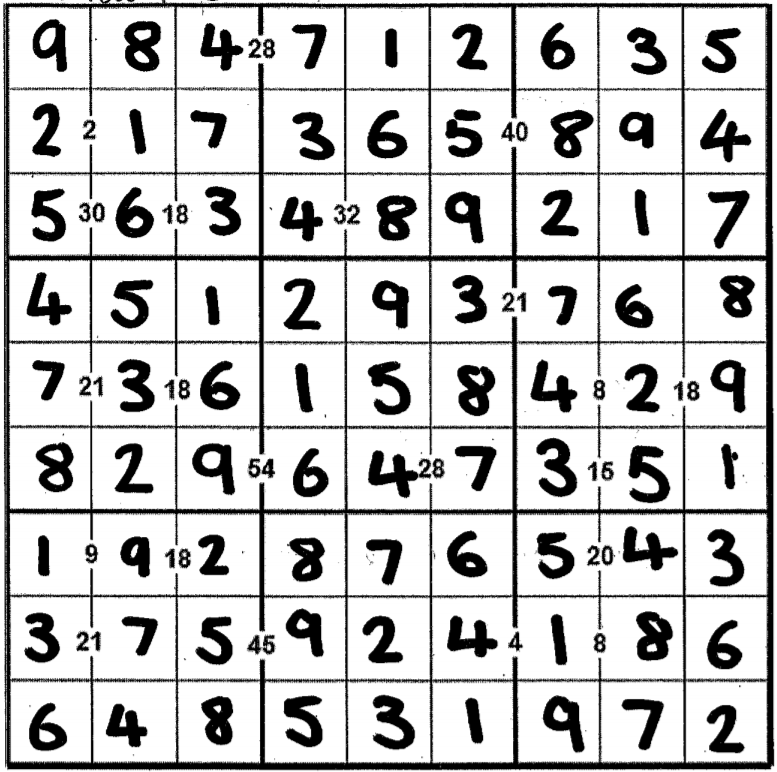#### You may also likeMake a set of numbers that use all the digits from 1 to 9, once and once only. Add them up. The result is divisible by 9. Add each of the digits in the new number. What is their sum? Now try some other possibilities for yourself!### Double Digit### Repeaters

Choose any 3 digits and make a 6 digit number by repeating the 3 digits in the same order (e.g. 594594). Explain why whatever digits you choose the number will always be divisible by 7, 11 and 13.

# Multiples Sudoku

##### Age 11 to 14 Challenge Level:

Thank you to everyone who sent in a solution. There were too many good solutions to mention everybody here, but this is a selection of what we received.

Most people said they began by using the clues that are the products of the numbers on their left and right. Spandan from MGIS Ahmedabad in India, George and James from Walton High School in the UK and Hannah and Amy from Priestlands School in the UK described listing factor pairs to help them. George and James said:

To start with, we listed all of the factors [of] the numbers in between two cells, provided they were both between 1 and 9 inclusive. We started with numbers with only one pair of factors, like 20, 21 and 54. This was made easier when we realised that some numbers that had two different factor possibilities could only have one solution because of all of the numbers around them that we had already filled in; some wouldn't work with the rest of the puzzle's rules. The cell with 8 in the middle could be 2 and 4 or 1 and 8. However, 2 was already in the same line.

Clara from Bangkok Patana School in Thailand, Alistair from King's School Grantham and Danielle from Greenfield Community College used common factors, and Clara also used some other properties of the numbers. Clara said:
I started off looking for prime and square numbers. 2 is a prime number so then one of the squares next to it had to be 2 and the other one 1. Then moving on to square numbers there was 9 and 4. You can not use the square root of these numbers because then the same number will be repeated twice which is one of the most important rules of sudoko. Therefore you must go with 1 and 9 (for an example) instead of 3 and 3.

If I had two answers that were next to each other such as the 9 and 18, I would see what common factors they had. In this case it was 9 and I knew that the two boxes next to 9 had to be either 1 or 9 as I said before. This then meant that I could put 9 between the 2 boxes and 1 and 2 on either side.

Daniella also used this method for some of the other numbers:
Then I did the 30 and 18 in the top left box. 5  6  3 (since the common multiples of 18 and 30 that are less than 10 are 3 and 6, and 30 as 3$\times$10 is not allowed).

Clara then said
:
After that, I looked for numbers with few factors such as 21. 21's factors are 1,3,7,21. I could not use 1 and 21 for this so I had to go for 3 and 7. I could also do this for many other larger numbers because many of them have only one set of factors where both numbers are under 10. After having found quite a few numbers I was able to solve the puzzle just as I would solve a normal sudoko.

This is Hannah and Amy's completed sudoku: